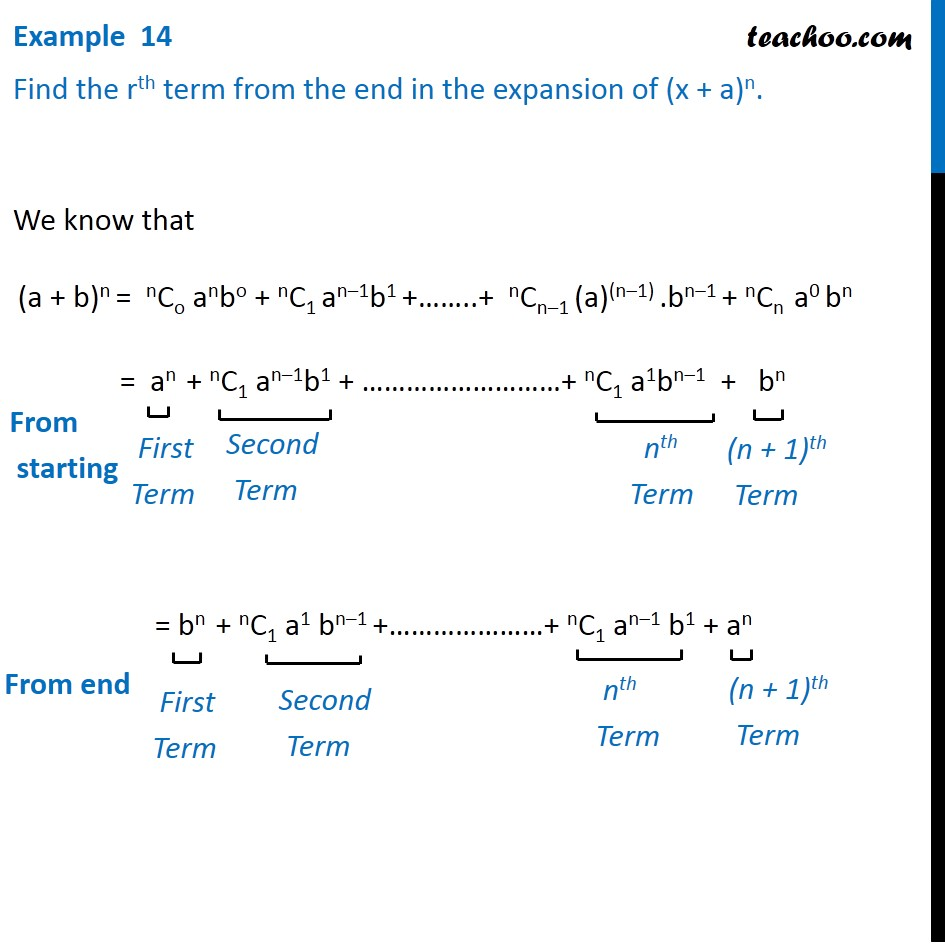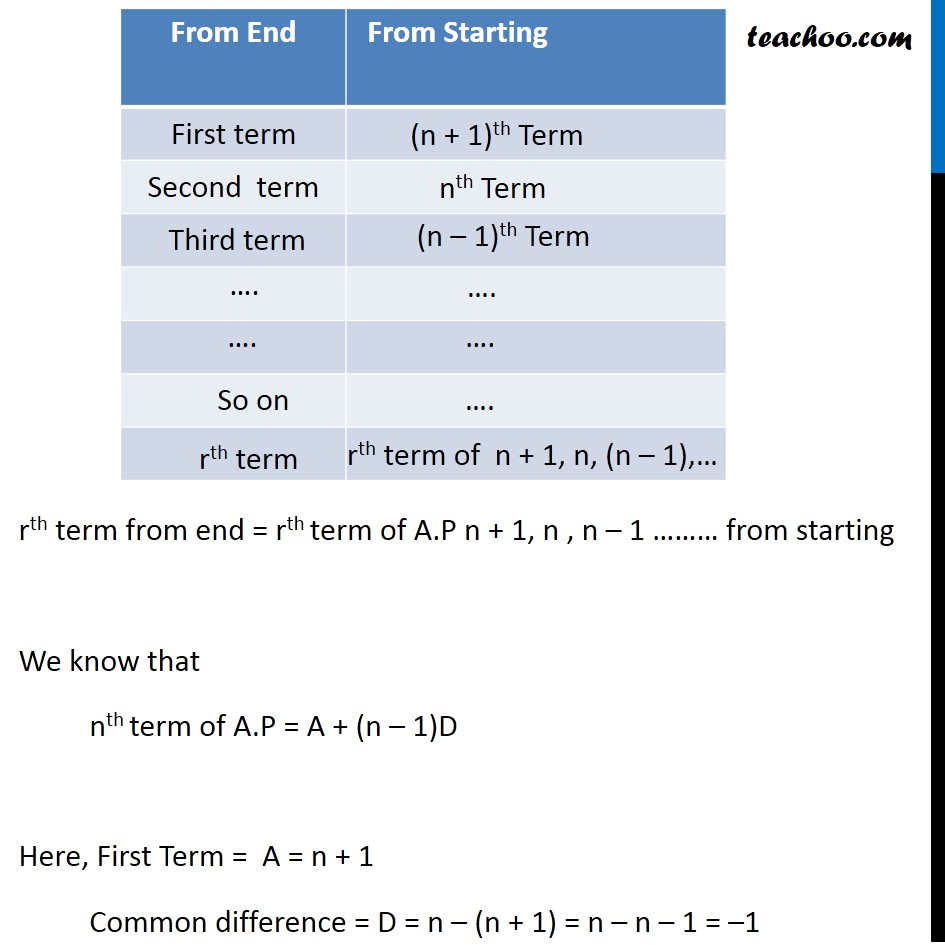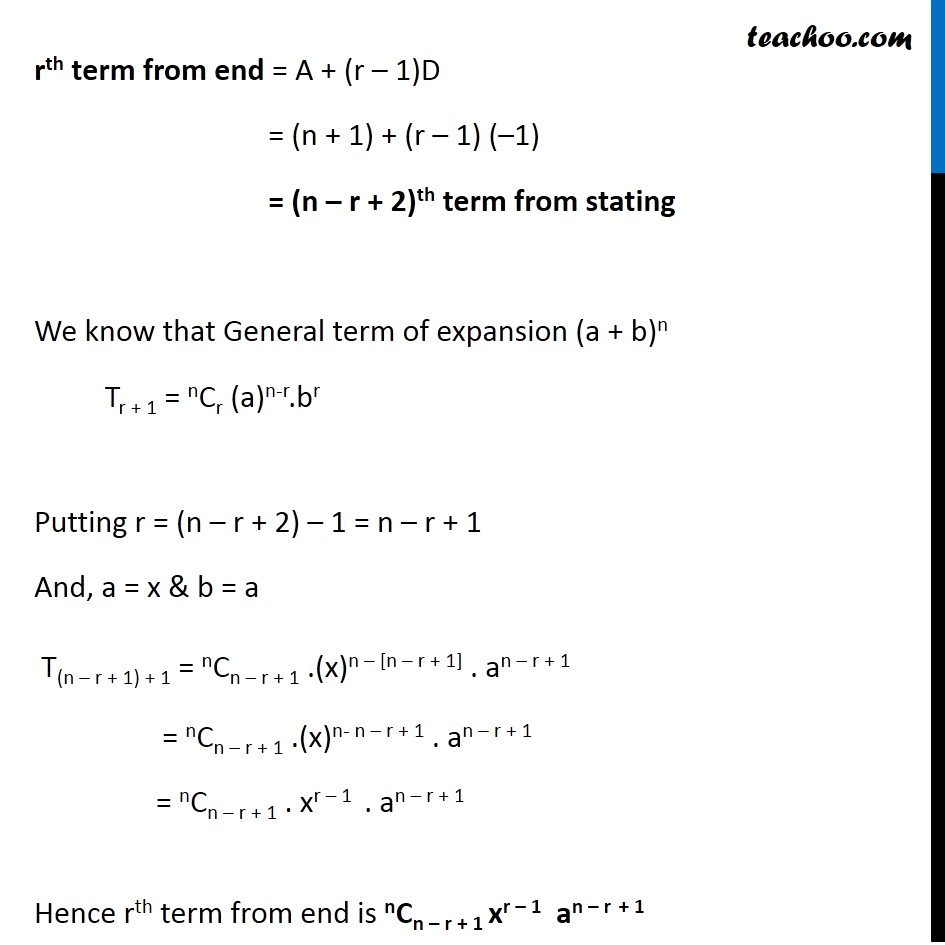Examples

Chapter 7 Class 11 Binomial Theorem
Serial order wiseLearn in your speed, with individual attention - Teachoo Maths 1-on-1 Class

### Transcript

Example 14 Find the rth term from the end in the expansion of (x + a)n. We know that (a + b)n = nCo anbo + nC1 an–1b1 +……..+ nCn–1 (a)(n–1) .bn–1 + nCn a0 bn = an + nC1 an–1b1 + ………………………+ nC1 a1bn–1 + bn = bn + nC1 a1 bn–1 +…………………+ nC1 an–1 b1 + an rth term from end = rth term of A.P n + 1, n , n – 1 ……… from starting We know that nth term of A.P = A + (n – 1)D Here, First Term = A = n + 1 Common difference = D = n – (n + 1) = n – n – 1 = –1 rth term from end = A + (r – 1)D = (n + 1) + (r – 1) (–1) = (n – r + 2)th term from stating We know that General term of expansion (a + b)n Tr + 1 = nCr (a)n-r.br Putting r = (n – r + 2) – 1 = n – r + 1 And, a = x & b = a T(n – r + 1) + 1 = nCn – r + 1 .(x)n – [n – r + 1] . an – r + 1 = nCn – r + 1 .(x)n- n – r + 1 . an – r + 1 = nCn – r + 1 . xr – 1 . an – r + 1 Hence rth term from end is nCn – r + 1 xr – 1 an – r + 1